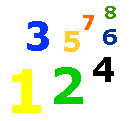# Making Change with Coins

Counting Coins - Making Change - Different Coins - Elementary Math Lessons & Tests - My Schoolhouse - Online Learning

 We can use coins to make different amounts of money.  Five pennies makes a nickel.  Two nickels make a dime.  Read  below as we discover ways to make change.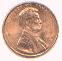1 penny, 1 cent, or 1� Count by one to count pennies. 1 cent, 2 cents, 3 cents, 4 cents and so on.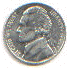1 nickel, 5 cents, or 5� Count by fives to count nickels. 5 cents, 10 cents, 15 cents, 20 cents and so on. 5 pennies = 1 nickel 1 cent + 1 cent + 1 cent + 1 cent + 1 cent = 5 cents or 1 nickel.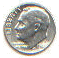1 dime, 10 cents, or 10� Count by tens to count dimes.  10 cents, 20 cents, 30 cents, 40 cents and so on. 10 pennies = 1 dime 1 cent + 1 cent + 1 cent + 1 cent + 1 cent + 1 cent + 1 cent + 1 cent + 1 cent + 1 cent = 10 cents or 1 dime. 2 nickels = 1 dime. 5 cents + 5 cents = 10 cents or one dime.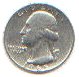1 quarter, 25 cents, or 25� Count by 25 to count quarters. 25 cents, 50 cents, 75 cents, \$1.00 and so on. 25 pennies = 1 quarter 5 nickels = 1 quarter 2 dimes and 1 nickel = 1 quarter   List the ways to make money. For example; 5� = 1or 5How many? List the ways to make...5� = 1 5� = 15� = 15� = 15� = 1 15� = 20� = 20� =

 How many? List the ways to make...25� = 25� = 1 25� = 2 25� = 1 1 25� = 2 5 25� = 25� = 25� = 15

 How many? List the ways to make...35� = 35� = 5 35� = 1 5 40� = 1 40� = 45� = 1 50� = 1 5 60� = 1

 Thank You for using My Schoolhouse